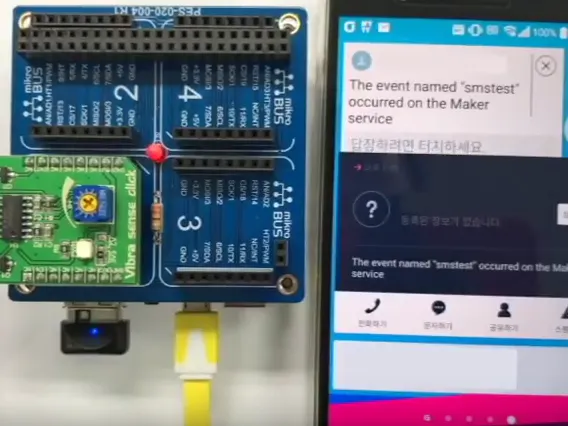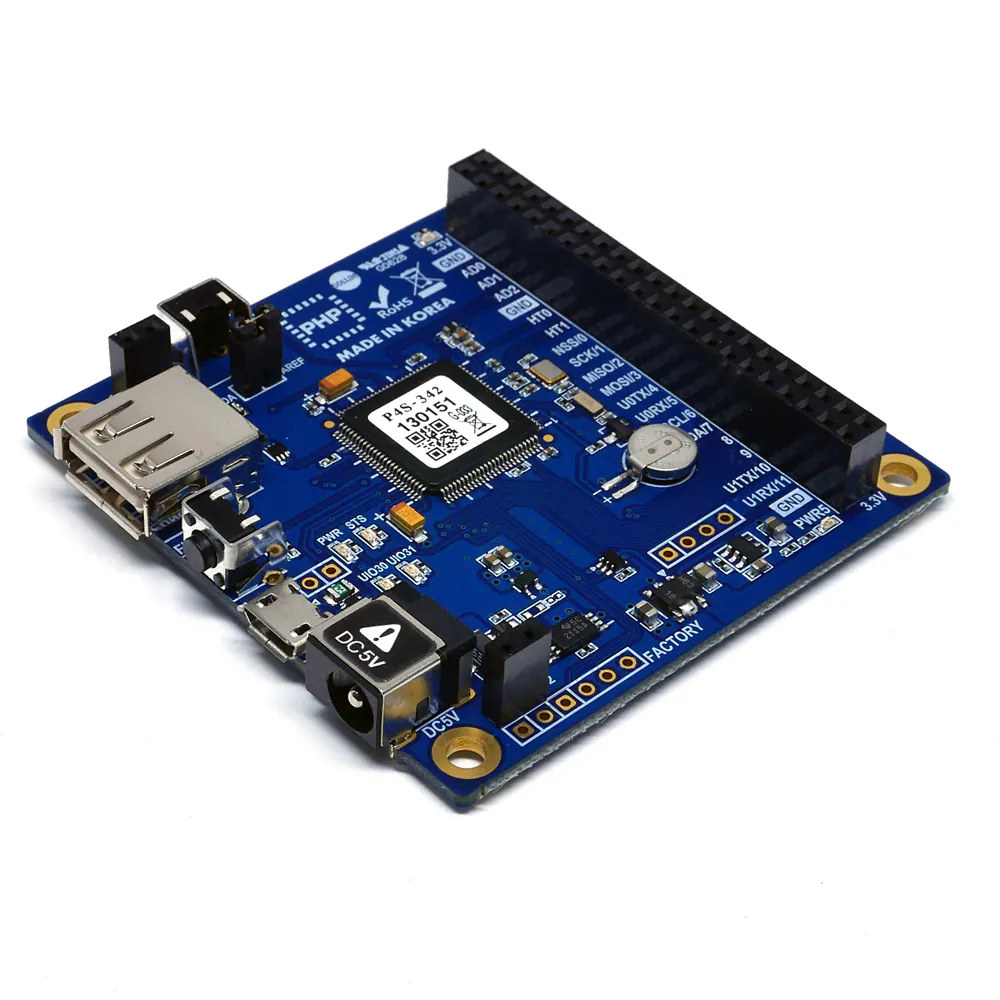# IFTTT's Android SMS Service with PHPoC Blue

This project shows how to use IFTTT Maker channel.

BeginnerShowcase (no instructions)8 hours1,073## Things used in this project

### Hardware componentsPHPoC Blue
×1PHPoC mikroBUS Expansion Board
×1
 MikroE Vibra Sense Click
×1

### Software apps and online servicesIFTTT Maker service

## Code

PHP
To fire IFTTT maker channel.
```<?php
include_once "/lib/sd_340.php";
include_once "ifttt.php";

echo "PHPoC example : IFTTT maker channel.\r\n";

define("PIN_EN", 12);
define("PIN_INT", 8);

uio_setup(0, PIN_INT, "in"); // INT
uio_setup(0, PIN_EN, "out"); // EN

uio_out(0, PIN_EN, 1);

\$previous_state = 0;
\$current_state = 0;

while(1)
{
\$current_state = uio_in(0, PIN_INT);
if(\$previous_state != \$current_state)
{
\$previous_state = \$current_state;
if(\$current_state == 1)
{
ifttt_maker("smstest", "", "", "");
}
}
}

?>
```

### ifttt.php

PHP
Send trigger message to IFTTT.
```<?php

include_once "/lib/sn_dns.php";

function ifttt_maker(\$event, \$value1, \$value2, \$value3)
{
\$host_name = "maker.ifttt.com";

\$tcp0_pid = pid_open("/mmap/tcp0");

pid_bind(\$tcp0_pid, "", 0);

for(;;)
{
\$state = pid_ioctl(\$tcp0_pid, "get state");

if(\$state == TCP_CLOSED)

if(\$state == TCP_CONNECTED)
break;
}

error_log("connected\r\n");

\$msg = "";
if(\$value1 != "" || \$value2 != "" || \$value3 != "")
{
\$msg = "{";

if(\$value1 != "")
\$msg .= "\"value1\" : \"".\$value1."\",";

if(\$value2 != "")
\$msg .= "\"value2\" : \"".\$value2."\",";

if(\$value3 != "")
\$msg .= "\"value3\" : \"".\$value3."\",";

\$msg = substr(\$msg, 0, strlen(\$msg) - 1);

\$msg .= " }";
}

\$http_req  = "POST /trigger/".\$event."/with/key/YOUR_KEY HTTP/1.1\r\n";
\$http_req .= "Host: \$host_name\r\n";
\$http_req .= "Content-Type: application/json\r\n";

if(strlen(\$msg) > 0)
\$http_req .= sprintf("Content-Length: %d", strlen(\$msg));

\$http_req .= "\r\n\r\n";

if(strlen(\$msg) > 0)
\$http_req .= \$msg;

error_log(\$http_req);

\$sent = pid_send(\$tcp0_pid, \$http_req);

\$rbuf = "";
for(;;)
{
\$temp = "";
\$rbuf .= \$temp;

if(strpos(\$rbuf, "\r\n\r\n") !== FALSE)
break;

if(pid_ioctl(\$tcp0_pid, "get state") == TCP_CLOSED || pid_ioctl(\$tcp0_pid, "get state") == SSL_CLOSED)
break;
}
pid_close(\$tcp0_pid);
error_log(\$rbuf);
error_log("\r\nconnection closed\r\n");
}
?>
```